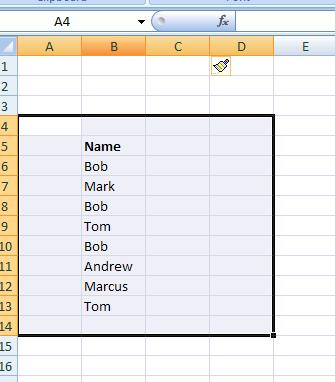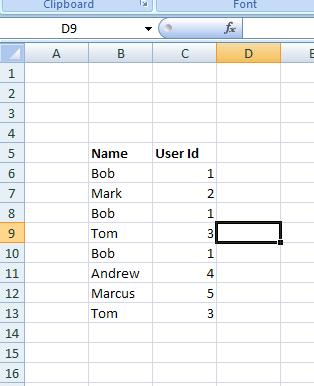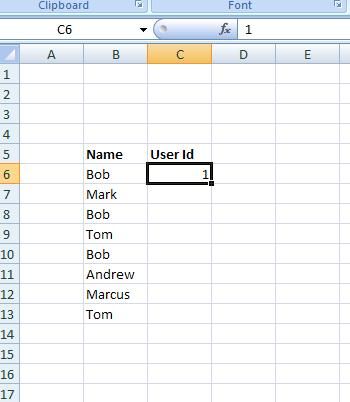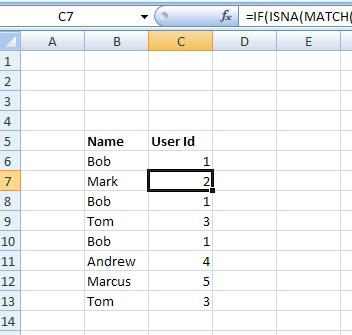Imagine that we have a list of names such as:And we want to give each one a unique identifier:So that the first name Bob has the identifier 1, and the next Mark has the identifier 2. This can be achieved by the following process.

We assign Bob (i.e the first person in the list) with an identifier 1:And then we insert the following formulae in the next cell below this and copy down:

=IF(ISNA(MATCH(B7,B6:\$B\$6,0)),MAX(C6:\$C\$6)+1,VLOOKUP(B7,B6:\$C\$6,2,FALSE))

So:The formulae works by looking for an occurrence of the name to date. If it doesn’t exist then it finds the maximum value of the identifier to date and adds one to give a new identifier. If a name does exist then a lookup is done to find the identifier for that name.

If more names are added at the bottom or in the middle and the formulae copies down, it still gives a distinct identifier.